## Asymptotic Curve

Given a Regular Surface, an asymptotic curve is formally defined as a curveonsuch that the Normal Curvature is 0 in the direction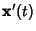for all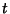in the domain of. The differential equation for the parametric representation of an asymptotic curve is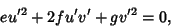(1)

where,, andare second Fundamental Forms. The differential equation for asymptotic curves on a Monge Patch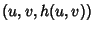is(2)

and on a polar patch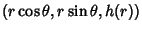is(3)

The images below show asymptotic curves for the Elliptic Helicoid, Funnel, Hyperbolic Paraboloid, and Monkey Saddle.Gray, A. Asymptotic Curves,'' Examples of Asymptotic Curves,'' Using Mathematica to Find Asymptotic Curves.'' §16.1, 16.2, and 16.3 in Modern Differential Geometry of Curves and Surfaces. Boca Raton, FL: CRC Press, pp. 320-331, 1993.